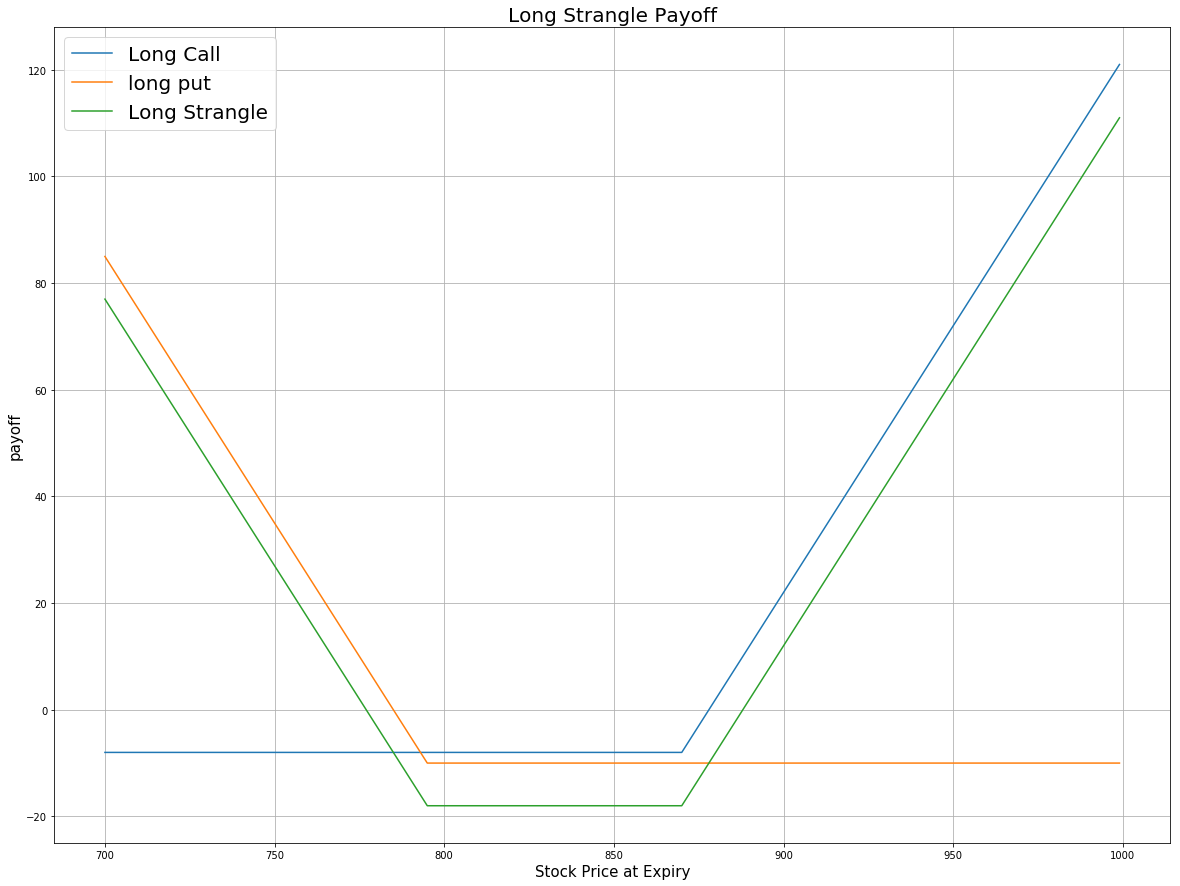# Option Strategies

## Strangle

### Introduction

Long Strangle is an Options trading strategy that consists of simultaneously buying an OTM put and an OTM call, where both contracts have the same underlying asset and expiration date. This strategy aims to profit from volatile movements in the underlying stock, either positive or negative.

Compared to a long straddle, the net debit of a long strangle is lower since OTM Options are cheaper. Additionally, the losing range of a long straddle is wider and the strike spread is wider.

### Implementation

Follow these steps to implement the long strangle strategy:

1. In the Initialize method, set the start date, end date, cash, and Option universe.
2. private Symbol _symbol;

public override void Initialize()
{
SetStartDate(2017, 4, 1);
SetEndDate(2017, 4, 30);
SetCash(100000);

_symbol = option.Symbol;
option.SetFilter(-5, 5, TimeSpan.FromDays(0), TimeSpan.FromDays(30));
}
def Initialize(self) -> None:
self.SetStartDate(2017, 4, 1)
self.SetEndDate(2017, 4, 30)
self.SetCash(100000)

self.symbol = option.Symbol
option.SetFilter(-5, 5, timedelta(0), timedelta(30))
3. In the OnData method, select the expiration date and strike prices of the contracts in the strategy legs.
4. public override void OnData(Slice slice)
{
if (Portfolio.Invested) return;

// Get the OptionChain
var chain = slice.OptionChains.get(_symbol, null);
if (chain == null || chain.Count() == 0) return;

// Select an expiration date
var expiry = chain.OrderBy(contract => contract.Expiry).Last().Expiry;

// Select the OTM call strike
var callStrikes = chain.Where(contract => contract.Expiry == expiry
&& contract.Right == OptionRight.Call
&& contract.Strike > chain.Underlying.Price)
.Select(contract => contract.Strike);
if (callStrikes.Count() == 0) return;
var callStrike = callStrikes.Min();

// Select the OTM put strike
var putStrikes = chain.Where(contract => contract.Expiry == expiry
&& contract.Right == OptionRight.Put
&& contract.Strike < chain.Underlying.Price)
.Select(contract => contract.Strike);
if (putStrikes.Count() == 0) return;
var putStrike = putStrikes.Max();
}
def OnData(self, slice: Slice) -> None:
if self.Portfolio.Invested: return

# Get the OptionChain
chain = slice.OptionChains.get(self.symbol, None)
if not chain: return

# Select an expiration date
expiry = sorted(chain, key=lambda contract: contract.Expiry, reverse=True).Expiry

# Select the OTM call strike
strikes = [contract.Strike for contract in chain if contract.Expiry == expiry]
call_strikes = [contract.Strike for contract in chain
if contract.Expiry == expiry
and contract.Right == OptionRight.Call
and contract.Strike > chain.Underlying.Price]
if len(call_strikes) == 0: return
call_strike = min(call_strikes)

# Select the OTM put strike
put_strikes = [contract.Strike for contract in chain
if contract.Expiry == expiry
and contract.Right == OptionRight.Put
and contract.Strike < chain.Underlying.Price]
if len(put_strikes) == 0: return
put_strike = max(put_strikes)
5. In the OnData method, call the OptionStrategies.Strangle method and then submit the order.
6. var optionStrategy = OptionStrategies.Strangle(_symbol, callStrike, putStrike, expiry);
Buy(optionStrategy, 1);
option_strategy = OptionStrategies.Strangle(self.symbol, call_strike, put_strike, expiry)
self.Buy(option_strategy, 1)

Option strategies synchronously execute by default. To asynchronously execute Option strategies, set the asynchronous argument to Falsefalse. You can also provide a tag and order properties to the Buy methods.

Buy(optionStrategy, quantity, asynchronous, tag, orderProperties);

self.Buy(option_strategy, quantity, asynchronous, tag, order_properties)


### Strategy Payoff

The payoff of the strategy is

$$\begin{array}{rcll} C^{OTM}_T & = & (S_T - K^{C})^{+}\\ P^{OTM}_T & = & (K^{P} - S_T)^{+}\\ P_T & = & (C^{OTM}_T + P^{OTM}_T - C^{OTM}_0 - P^{OTM}_0)\times m - fee \end{array}$$ $$\begin{array}{rcll} \textrm{where} & C^{OTM}_T & = & \textrm{OTM call value at time T}\\ & P^{OTM}_T & = & \textrm{OTM put value at time T}\\ & S_T & = & \textrm{Underlying asset price at time T}\\ & K^{C} & = & \textrm{OTM call strike price}\\ & K^{P} & = & \textrm{OTM put strike price}\\ & P_T & = & \textrm{Payout total at time T}\\ & C^{OTM}_0 & = & \textrm{OTM call value at position opening (debit paid)}\\ & P^{OTM}_0 & = & \textrm{OTM put value at position opening (debit paid)}\\ & m & = & \textrm{Contract multiplier}\\ & T & = & \textrm{Time of expiration} \end{array}$$

The following chart shows the payoff at expiration:The maximum profit is unlimited if the underlying price rises to infinity at expiration.

The maximum loss is the net debit paid, $C^{OTM}_0 + P^{OTM}_0$. It occurs when the underlying price at expiration is the same as when you opened the trade. In this case, both Options expire worthless.

### Example

The following table shows the price details of the assets in the algorithm at Option expiration (2017-04-22):

AssetPrice ($)Strike ($)
Call8.80835.00
Put9.50832.50
Underlying Equity at expiration843.19-

Therefore, the payoff is

$$\begin{array}{rcll} C^{OTM}_T & = & (S_T - K^{C})^{+}\\ & = & (843.19-835.00)^{+}\\ & = & 8.19\\ P^{OTM}_T & = & (K^{P} - S_T)^{+}\\ & = & (832.50-843.19)^{+}\\ & = & 0\\ P_T & = & (C^{OTM}_T + P^{OTM}_T - C^{OTM}_0 - P^{OTM}_0)\times m - fee\\ & = & (8.19+0-8.80-9.50)\times100-1.00\times2\\ & = & -1013 \end{array}$$

So, the strategy losses \$1,013.

The following algorithm implements a long strangle Option strategy:

You can also see our Videos. You can also get in touch with us via Discord.